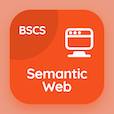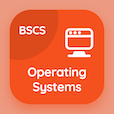Computer Science Courses Online

Digital Logic Design Practice Tests

Digital Logic Design Online Tests

The Book Axiomatic Definition of Boolean Algebra Multiple Choice Questions (MCQ Quiz) with answers, Axiomatic Definition of Boolean Algebra MCQ Quiz PDF download to study online logic design degree courses. Study Boolean Algebra and Logic Gates Multiple Choice Questions and Answers (MCQs), Axiomatic Definition of Boolean Algebra quiz answers PDF for BSc computer science. The e-Book Axiomatic Definition of Boolean Algebra MCQ App Download: canonical and standard forms, basic definition of boolean algebra, boolean functions in dld, basic theorems and properties of boolean algebra test prep for software engineering online courses.

The MCQ: Boolean algebra is also called PDF, "Axiomatic Definition of Boolean Algebra" App Download (Free) with switching algebra, arithmetic algebra, linear algebra, and algebra choices for BSc computer science. Practice axiomatic definition of boolean algebra quiz questions, download Amazon eBook (Free Sample) for software engineering online courses.

## Logic Design MCQs: Axiomatic Definition of Boolean Algebra Quiz Questions

MCQ: Boolean algebra is also called

A) switching algebra
B) arithmetic algebra
C) linear algebra
D) algebra

MCQ: Boolean algebra is defined as a set of

A) three values
B) two values
C) four values
D) five values

MCQ: Boolean algebra is an algebraic structure with two arithmetic operations

B) subtraction and multiplication

MCQ: An identity element w.r.t addition

A) x-1
B) x+1
C) x-0
D) x+0

MCQ: In Boolean algebra, the OR operation is performed by which of the following properties?

A) associative properties
B) commutative properties
C) distributive properties
D) identity element

### Practice Tests: Digital Logic Design Exam Prep

Download Digital Logic Design Quiz App, Semantic Web MCQ App, and Operating Systems MCQs App to install for Android & iOS devices. These Apps include complete analytics of real time attempts with interactive assessments. Download Play Store & App Store Apps & Enjoy 100% functionality with subscriptions!